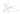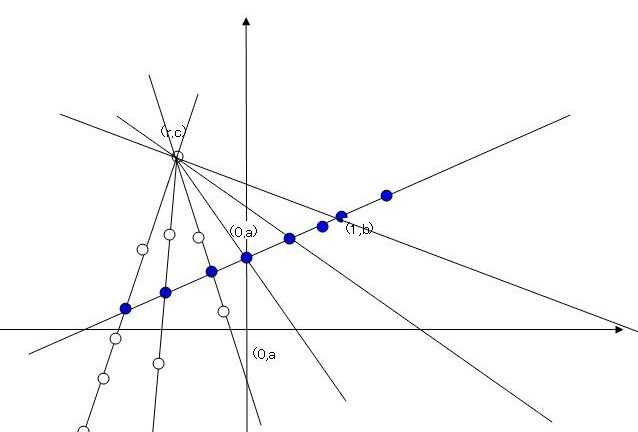## 函数方程之无限维解

Wed, 22nd January 2020Edit on Githubfunctionlinear

# 问题溯源

2009年1月northwolves提问
$f(x)$ 定义在实数域上，且$f(x+y)+f(x-y)-2f(x)-2y^2=0$ ，求$f(x)$

# 初步进展

mathe提议, 将$g(x)=f(x)-x^2$代入。
northwolves然后使用这种方法进一步推导得出:
$f(x+y)+f(x-y)-2f(x)-2y^2=0$.
Let $g(x)=f(x)-x^2$,得出
$g(x+y)+(x+y)^2+g(x-y)+(x-y)^2-2g(x)-2x^2-2y^2=0$
$g(x+y)+g(x-y)-2g(x)=0$
shshsh_0510接下去指出g(x)可以是任意线性函数,即$g(x)=ax+b$， 所以
$f(x)=x^2+ax+b$,其中$a,b \in R$.

# 解析解

$f^{\prime}(x+y)=f^{\prime}(x)+2y$.

# 是否还有其它解

shshsh_0510继续提问，如何说明
1）H存在性的证明？
2）是否还有其他解？

mathe回复
H存在性需要假设选择公理成立，这个时候就可以使用佐恩引理来证明H的存在性。

$x=ny$代入，可以得到
$g((n+1)y)-g(ny)=g(ny)-g((n-1)y)=\dots=g(y)-g(0)=g(y)$

$y=\frac tm$，其中m为另外一个整数，得到$g(\frac nm t)=\frac nm g(t)$

$\mathcal{R}$为所有$R$中那些满足任意有限个元素的有理线性组合都不是0的集合构成的集簇。

shshsh_0510为这个过程配了一个示意图:# 类似问题分析

wayne在他的微信群里面发过另外一个类似的函数方程：

mathe分析结果是只有唯一情况就是$a\diamond b=ab$, 但是他很好奇如果最后的不等式条件被去除后结果会如何。分析结果表明，$a\diamond b=a f(b)$, 其中函数$f(x)=\exp(g(\ln(x)))$,其中$g(x)$和前面问题的$g(x)$完全相同，是R在Q中的任意线性函数

1. 证明左消去律: $a\diamond b=a\diamond c => b=c$
2. 右消去律: $a\diamond c=b\diamond c => a=b$
3. 右单位元: $a\diamond 1 = a$
4. 左乘性: $b\diamond a = b(1\diamond a)$
5. 三元交换: $a\diamond(b\diamond c)=c\diamond(b\diamond a)$
6. 倒数率： $1\diamond \frac 1 a =\frac1{1\diamond a}$
定义$f(x)=1\diamond x$后，可以得出
7. $f(xf(y))=yf(x)$
8. $f(f(y))=y$
9. $f(xz)=f(x)f(z)$

$f(x+2f(y))=f(x+2y_1-y_2)=f(x_1+2y_1+x2-y_2)=x_1+2y_1-\frac12 x_2+\frac12 y_2$
$f(x)+y+f(y)=f(x_1+x_2)+y_1+y_2+f(y_1+y_2)=x_1-\frac12 x_2+y_1+y_2+y_1-\frac12 y_2=x_1+2y_1-\frac12 x_2+\frac12 y_2$

Github